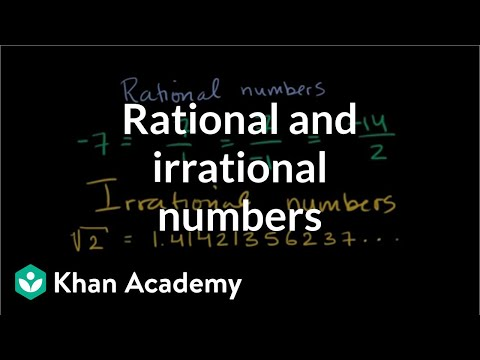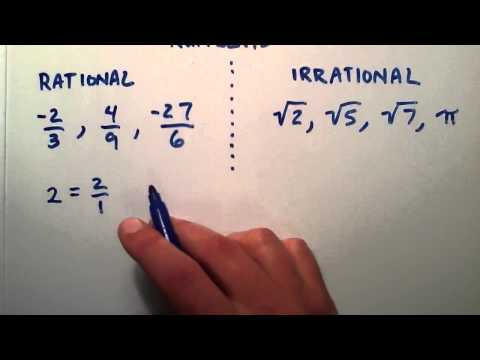## Rational and Irrational Numbers

Subject: Compulsory Maths

#### Overview

A number that can be made by dividing two numbers is called rational number. The numbers which are not rational and that cannot be made by dividing two integers are called irrational numbers. The sets of number system i.e Natural numbers, Whole numbers, Integers, Rational numbers and Irrational numbers are defined under the set of real number system.
##### Rational and Irrational Numbers

Rational Numbers

In general, A number that can be made by dividing two numbers is called rational number. Any numbers which can be expressed in the form of $\frac{a}{b}$ where a and b are integers and b ≠ 0 are called rational numbers. The set of rational numbers is denoted by the letter 'Q'. For examples Q = ( $\frac{1}{2}$, $\frac{3}{4}$, $\frac{212}{100}$, $\frac{20}{12}$, etc.. )

The set of the rational number is the set that includes the sets of natural numbers (N), whole numbers (W), and integers (Z). Therefore, the sets of natural numbers, whole numbers and integers are the proper subsets of the set of rational number.∴N < Q, W < Q and Z < Q.Terminating and Non-Terminating rational numbers

When the rational number gets decimalised, the obtained decimal may be terminating or non-terminating decimal. Terminating rational number as decimal generally comes to an end and doesn't repeat after decimal. For example $\frac{1}{2}$ = 0.5, $\frac{1}{4}$ = 0.25, $\frac{3}{8}$ = 0.375 etc.) are the examples of terminating decimal.

Non-Terminating rational number as decimal goes on repeating in a pattern after the decimal. For examples ( $\frac{1}{3}$ = 0.333333..., $\frac{1}{15}$ = 0.0666666.... etc. are non-terminating rational number as decimal.

Irrational Numbers

The numbers which are not rational and that cannot be made by dividing two integers are called irrational numbers . If the irrational numbers are decimalised, the decimals are non-terminating non-recurring that goes on forever without repeating. For example ( $\sqrt{5}$ = 2.2360679775,( $\sqrt{2}$ = 1.41423562 etc. are the examples of irrational numbers.

Real Number System

The sets of number system i.e Natural numbers, Whole numbers, Integers, Rational numbers and Irrational numbers are defined under the set of real number system. The following chart shows the real number system :-

##### Things to remember
• A  number that can be made by dividing two numbers is called rational number.
• The numbers which are not rational and that cannot be made by dividing two integers are called irrational numbers .
• It includes every relationship which established among the people.
• There can be more than one community in a society. Community smaller than society.
• It is a network of social relationships which cannot see or touched.
• common interests and common objectives are not necessary for society.
##### Videos for Rational and Irrational Numbers##### Introduction to rational and irrational numbers | Algebra I | Khan Academy##### What is the Difference Between Rational and Irrational Number

In general, A number that can be made by dividing two numbers is called rational number. Any numbers which can be expressed in the form of $\frac{a}{b}$ where a and b are integers and b ≠ 0 are called rational numbers.

The set of rational numbers is denoted by the letter 'Q'.

0=$\frac{0}{1}$ . It is in $\frac{a}{b}$ form . So ,0 is a rational number .

-8 =-$\frac{8}{1}$ . It is  in $\frac{a}{b}$ form . So, -8 is a rational number .

0.25= It is terminating decimal . So, 0.25 is a rational number .

0.16  it is non-terminating recurring decimal . So, 0.16 is a rational number .

Rational number at the middle of 2 and 3 = $\frac{1}{2}$(2+3)=$\frac{5}{2}$

Rational number at the middle of 2 and $\frac{5}{2}$ = $\frac{1}{2}$ (2+$\frac{5}{2}$ = $\frac{1}{2}$ x $\frac{9}{2}$ =$\frac{9}{4}$

So , $\frac{5}{2}$ and $\frac{9}{4}$ are any two rational numbers between 2 and 3.